# How much privacy do “Privacy Zones” provide?

Alexandra Kapp

In this post, I gave an overview of different privacy-enhancing techniques for mobility data. This post is the first to deep-dive into single specific techniques by looking into privacy zones.

Social-network sports apps like Strava are used to track and share personal sporting performances, including pictures and GPS traces. This has led to undesired events: for example, in 2018, bikes worth more than \$20,000 were stolen from a guy who had posted pictures of all his bikes on Strava in addition to his bike trips that started and ended at his house. This allowed the thief to easily determine at which house a bunch of expensive bikes were stored.

To protect sensitive locations such as the home location, some of those sports app providers like Garmin or Strava offer a privacy zone functionality. Users can define sensitive locations around which a privacy zone is created according to a preferred radius (see Figure 1 for an example of the privacy zone user interface by Garmin). Any shared routes will be cut off at the boundary of the privacy zone (see Figure 2).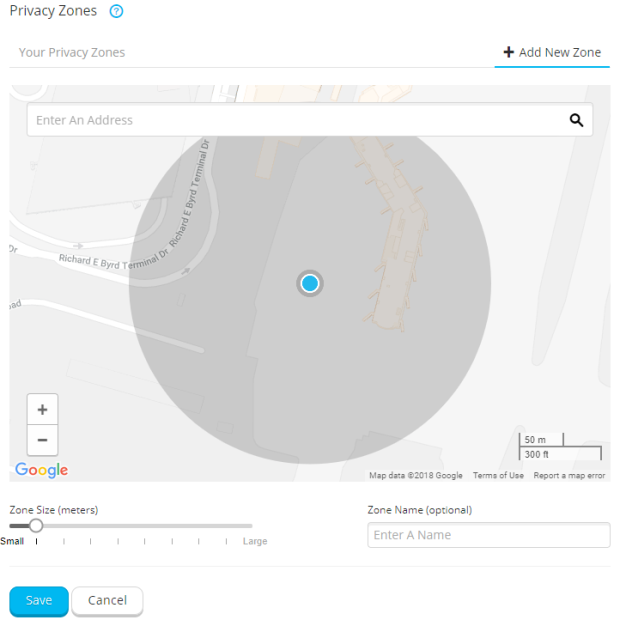Figure 1: Example screenshot by Garmin that shows the user interface for the privacy zone functionality

This method has obvious limitations: in such a basic implementation where all trajectories end at the boundary of the privacy zone which is a perfect circle, it only needs a few points to be able to reconstruct the center of that circle, i.e., the sensitive location.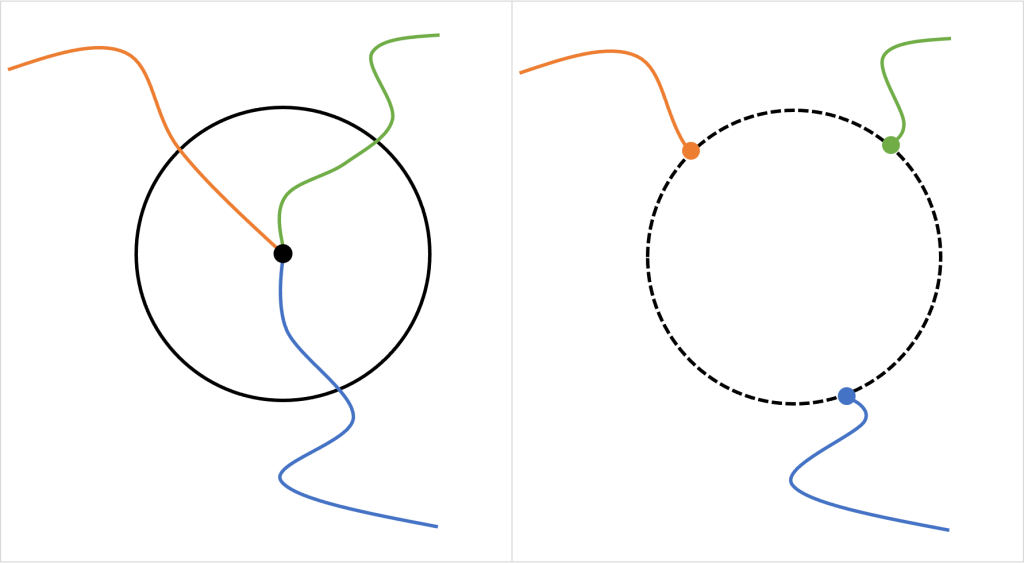Figure 2: An example of a privacy zone: Left: three routes lead to a location that has been defined as a sensitive location (e.g., home location). The circle indicates the privacy zone around the sensitive location. Right: all routes are cut off at the boundary of the circle. The figure already suggests that the circle, and thus the center of the circle, can be reconstructed given the shortened trajectories.

Hassan et al. (2018) show how such centers can easily be computed mathematically from Strava data while Mink et al. (2022) showed with a user study that no such mathematical capabilities are even necessary, as for the most common privacy zone size, 68% of guesses (i.e., estimating based on the visual map representation) fell within 50 meters of the hidden location when participants were shown just 3 activities.

We can conclude, that privacy zones in their basic implementation do not provide satisfying privacy.

### Can Privacy Zones Be Improved?

Hassan et al. (2018) test three different obfuscation techniques to modify privacy zones and compare how much the location reconstruction success is reduced.

1. Modify radius size: Enlarge the size of the radius (see Figure 3). According to the authors’ evaluation, this has no real privacy improvement. Only, when the radius was enlarged to such an extent, that a substantial number of trajectories are removed completely, thus highly sacrificing utility.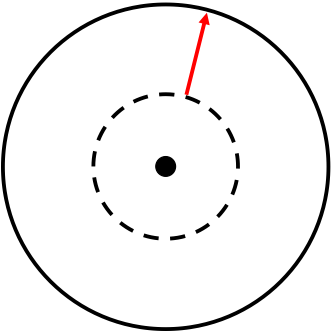Figure 3: 1) Modify radius. The privacy zone radius is enlarged.

2. Fuzzy intersection points: Not the exact intersection with the boundary of the privacy zone is used to cut trajectories, instead, a random number of GPS points is removed additionally (see Figure 4). For a radius of ~400 meters (0.250 miles) and a fuzz value of 40 meters (i.e., the maximum distance removed additionally) the accuracy of correctly determining the sensitive location drops from 96% (without fuzzing) to 32%. For a fuzz value of 200 meters, it drops down to 15%. Thus, the authors conclude that this method can significantly increase privacy, at the same time, the authors note that there are likely more expressive attack models to overcome fuzzing noise than the basic one they used.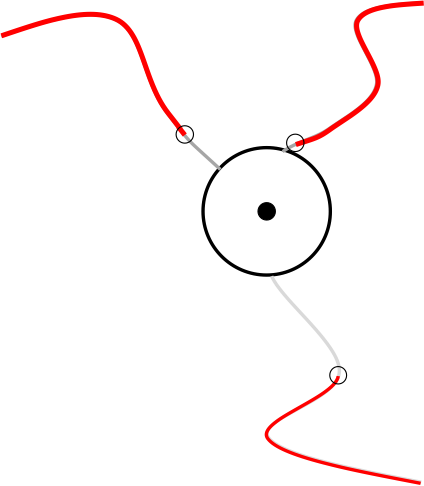Figure 4: 2) Fuzzy intersection points. The grey lines are removed from the trajectories in addition to the part that lies within the privacy zone.

3. Spatial cloaking by shifting the center: By shifting the center of the privacy zone, a reconstruction of the center will not reveal the true sensitive location. Of course, the true sensitive location still needs to be within the shifted privacy zone (see Figure 5).

For example, the OpenBikeSensor makes use of such an implementation.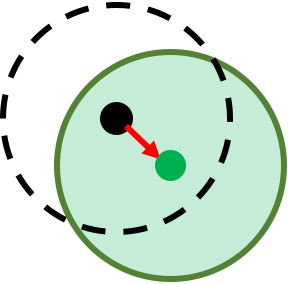Figure 5: Spatial cloaking by shifting the center. The dotteld line is the original privacy zone and the green circle is the shifted privacy zone.

For this method, the authors run a different attack, because determining the center of the privacy zone is no longer revealing sensitive information. Instead, they use the direction of the trajectories (based on the last two points) as an attack vector: by extending the trajectory (with a straight line) they determine where the trajectories cross paths. The middle of all crossings is used as a prediction and it is considered true if it falls within 50 meters of the true location (see Figure 6). Even though this is a very simple attack implementation that only takes the two last points of a trajectory to determine the direction and draws a straight light to determine the crossing, they have a success rate of 41.3% for a radius of ~400 meters (0.250 miles).

Both obfuscation methods, fuzzy intersection points and shifting the center, increase privacy and significantly increase the complexity of the attack. Depending on the use case and the attacker this might provide sufficient protection in practice. Still, it should be noted that such mechanisms can be broken with a bit of effort to a non-negligible success rate.

Dieser Text erschien zuerst auf Alexandras privatem Blog.

Zur Blogübersicht